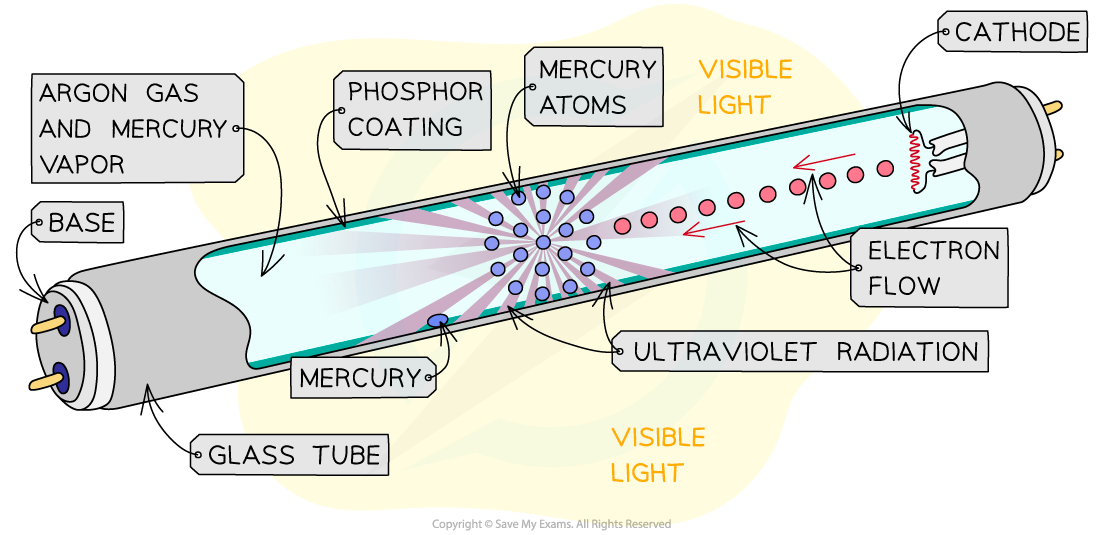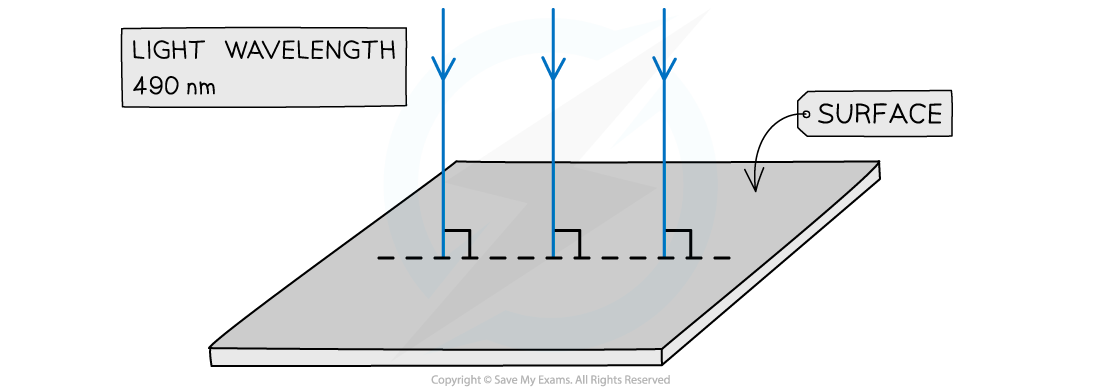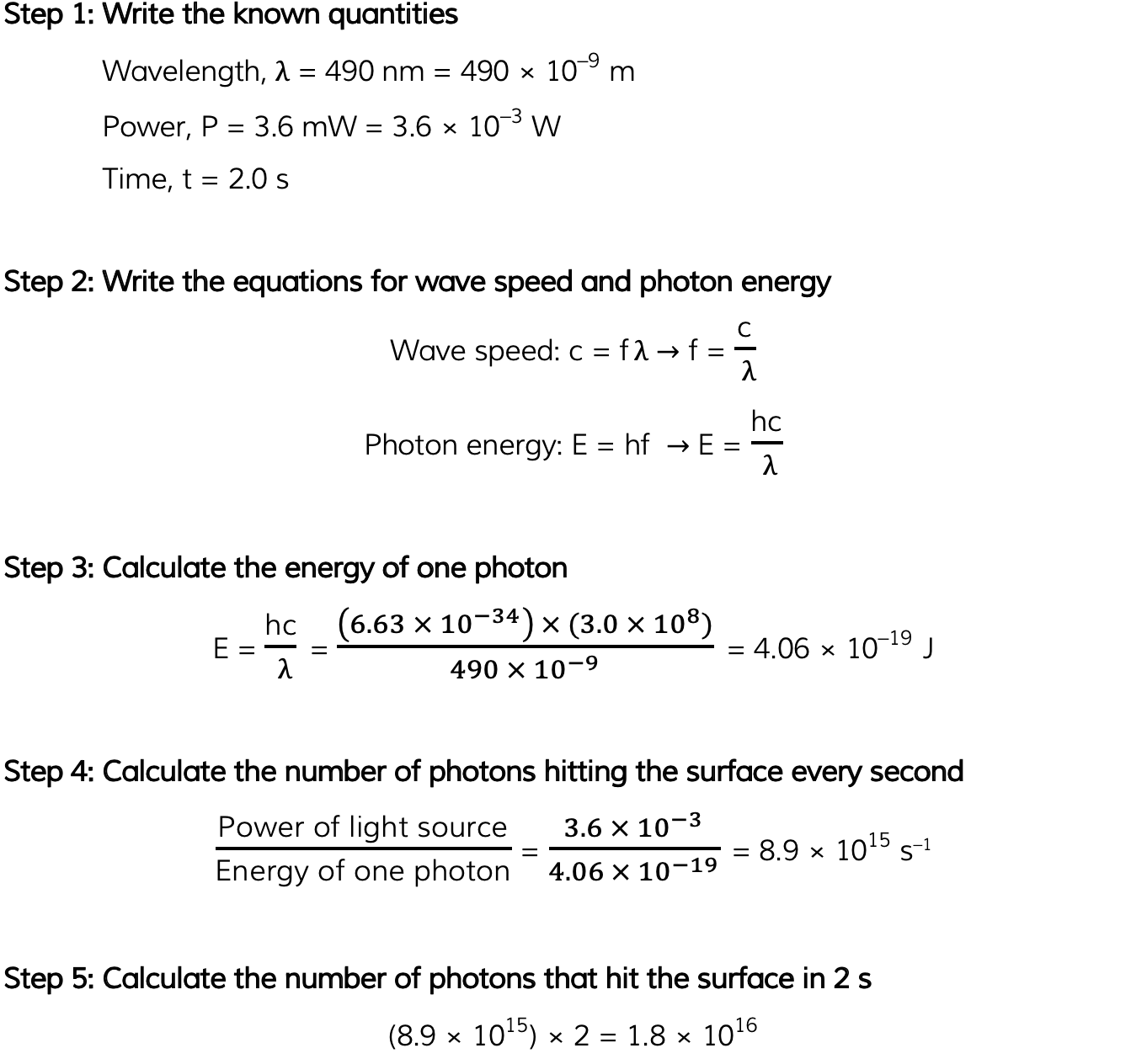# AQA A Level Physics复习笔记2.5.1 Collisions of Electrons with Atoms

### Ionisation & Excitation

• Ionisation of an atom is:

The removal, or addition, of an electron from, or to, an atom when given sufficient energy

• Excitation of electrons is:

When an electron is given enough energy to move up an energy level, but not enough to leave the atom

#### Fluorescent Tube

• Fluorescence occurs when an electron in an atomic orbital absorbs energy from an interaction with a photon or a collision with another atom
• Fluorescent tubes are partially evacuated glass tubes filled with low-pressure mercury vapour with a phosphor coating on the glass
• When an electric current is passed through the vapour, the electrons in mercury are excited and move to a higher energy level
• This high energy level state is unstable and so the electron moves back to its original state, or de-excites
• As it de-excites, the electron releases some of that energy in the form of a UV photon
• This UV light then excites the electrons in the phosphor coating
• As a result, visible light photons are released when the electrons return to their original energy state, which provides the fluorescent glowFluorescent tubes operate on the basis of excitation and de-excitation of electrons leading to the emission of visible light

### The Photon Model

• Photons are fundamental particles which make up all forms of electromagnetic radiation
• A photon is a massless “packet” or a “quantum” of electromagnetic energy
• What this means is that the energy is not transferred continuously, but as discrete packets of energy
• In other words, each photon carries a specific amount of energy, and transfers this energy all in one go, rather than supplying a consistent amount of energy

#### Calculating Photon Energy

• The energy of a photon can be calculated using the formula:

E = hf

• Using the wave equation, energy can also be equal to:• Where:
• E = energy of the photon (J)
• h = Planck's constant (J s)
• c = the speed of light (m s-1)
• f = frequency (Hz)
• λ = wavelength (m)

• This equation tells us:
• The higher the frequency of EM radiation, the higher the energy of the photon
• The energy of a photon is inversely proportional to the wavelength
• A long-wavelength photon of light has a lower energy than a shorter-wavelength photon

#### Worked Example

Light of wavelength 490 nm is incident normally on a surface, as shown in the diagram.The power of the light is 3.6 mW. The light is completely absorbed by the surface.Calculate the number of photons incident on the surface in 2.0 s.#### Exam Tip

Make sure you learn the definition for a photon: discrete quantity / packet / quantum of electromagnetic energy are all acceptable definitions.The values of Planck’s constant and the speed of light will always be available on the datasheet, however, it helps to memorise them to speed up calculation questions!